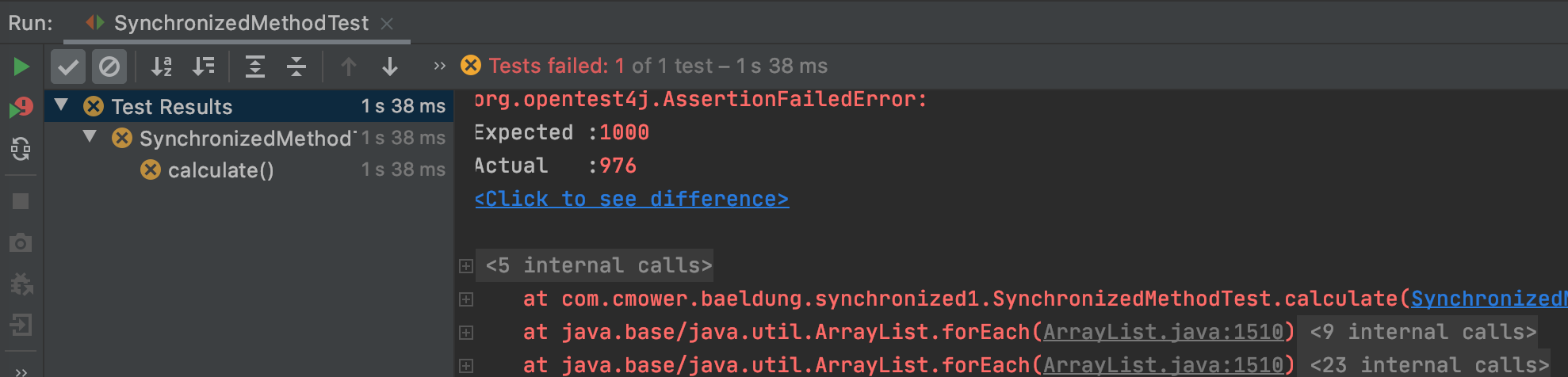# 博客之星：我去，你竟然还不会用 synchronized

• 2021 年 11 月 11 日
• 本文字数：1929 字

阅读完需：约 6 分钟

class SynchronizedMethodTest {

@Test

void calculate() {

}

}

calculate() 方法上会有一个 @Test 的注解，表示这是一个测试方法。添加具体的代码，如下所示：

SynchronizedMethod summation = new SynchronizedMethod();

IntStream.range(0, 1000)

.forEach(count -> service.submit(summation::calculate));

service.awaitTermination(1000, TimeUnit.MILLISECONDS);

assertEquals(1000, summation.getSum());

1）Executors.newFixedThreadPool() 方法可以创建一个指定大小的线程池服务 ExecutorService。

2）通过 IntStream.range(0, 1000).forEach() 来执行 calculate() 方法 1000 次。

3）通过 assertEquals() 方法进行判断。### 02、synchronized 的用法

1）直接用在方法上，就像下面这样：

public synchronized void synchronizedCalculate() {

setSum(getSum() + 1);

}

@Test

void synchronizedCalculate() throws InterruptedException {

SynchronizedMethod summation = new SynchronizedMethod();

IntStream.range(0, 1000)

.forEach(count -> service.submit(summation::synchronizedCalculate

【一线大厂Java面试题解析+后端开发学习笔记+最新架构讲解视频+实战项目源码讲义】浏览器打开：qq.cn.hn/FTf 免费领取

));

service.awaitTermination(1000, TimeUnit.MILLISECONDS);

assertEquals(1000, summation.getSum());

}

2）用在 static 方法上，就像下面这样：

public class SynchronizedStaticMethod {

public static int sum;

public synchronized static void synchronizedCalculate() {

sum = sum + 1;

}

}

sum 是一个静态变量，要修改静态变量的时候，就需要把方法也变成 static 的。

class SynchronizedStaticMethodTest {

@Test

void synchronizedCalculate() throws InterruptedException {

IntStream.range(0, 1000)

.forEach(count -> service.submit(SynchronizedStaticMethod::synchronizedCalculate));

service.awaitTermination(1000, TimeUnit.MILLISECONDS);

assertEquals(1000, SynchronizedStaticMethod.sum);

}

}

synchronized static 和 synchronized 不同的是，前者锁的是类，同一时间只能有一个线程访问这个类；后者锁的是对象，同一时间只能有一个线程访问方法。

3）用在方法块上，就像下面这样：

public void synchronisedThis() {

synchronized (this) {

setSum(getSum() + 1);

}

}

public static void synchronisedThis() {

synchronized (SynchronizedStaticMethod.class) {

sum = sum + 1;

}

}

@Test

void synchronisedThis() throws InterruptedException {

SynchronizedMethod summation = new SynchronizedMethod();

IntStream.range(0, 1000)

.forEach(count -> service.submit(summation::synchronisedThis));

service.awaitTermination(1000, TimeUnit.MILLISECONDS);

assertEquals(1000, summation.getSum());

}

## 评论